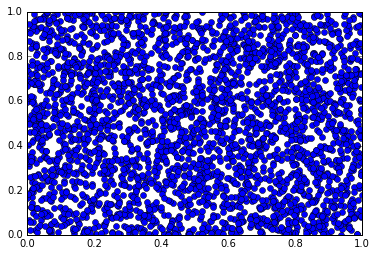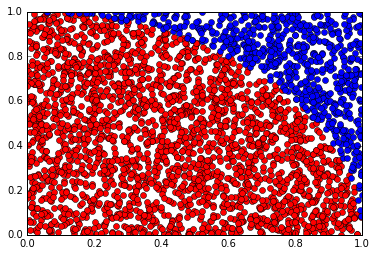# Computing $\pi$ using Sampling¶

In :
import numpy as np
import matplotlib.pyplot as plt

In :
nsamples = 3000


Let's start with a uniform distribution on the unit square $[0,1]^2$.

Create a 2D array samples of shape (2, nsamples):

In :
samples = np.random.rand(2, nsamples)


Plot them:

In :
plt.plot(samples, samples, "o")

Out:
[<matplotlib.lines.Line2D at 0x7ff41d4310f0>]Now we would like to find $\int_[0,1\\int_[0,1] p(x,y) dx dy$ where $p(x,y)$ is 1 if $(x,y)$ is in the unit circle around the origin, otherwise zero.

$p(x)$ is not a probability distribution. (But with a scaling factor $\alpha$ it can be one, so that

$$\frac1{\alpha} \int_{[0,1]}\int_{[0,1]} p(x,y) dx dy=1.$$

So it is $\alpha$ we're looking for. Then:

$$\frac1{\alpha} \int_{[0,1]}\int_{[0,1]} p(x,y) dx dy=E=\frac1{\alpha} \sum_{i=1}^N p(x_i)$$

Next, evaluate $p$ for each sample:

In :
p = np.zeros(nsamples)

r = samples**2 + samples**2
p[r<=1] = 1


Plot the value of $p$ for the samples:

In :
plt.plot(samples[0, p==1], samples[1, p==1], "o", color="red")
plt.plot(samples[0, p==0], samples[1, p==0], "o", color="blue")

Out:
[<matplotlib.lines.Line2D at 0x7ff41bf95160>]Evaluate $\alpha$:

In :
alpha = np.sum(p) / nsamples
alpha

Out:
0.78133333333333332
In :
my_pi = 4*alpha
my_pi

Out:
3.1253333333333333
In [ ]: